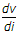# Electronics and Communication Engineering - Electronic Devices and Circuits

46.

Assertion (A): Intrinsic semiconductor is an insulator at 0 K.

Reason (R): Fermi level in intrinsic semiconductor is in the centre of forbidden energy band.

 A. Both A and R are true and R is correct explanation of A B. Both A and R are true but R is not a correct explanation of A C. A is true but R is false D. A is false but R is true

Explanation:

No answer description available for this question. Let us discuss.

47.

Typical values of h parameters at about 1 mA collector current for small signal audio amplifier in CE configuration are :

 A. hie = 100 Ω, hre = 10-1, hfe = 50, hoe = 1 m mho B. hie = 5 kΩ, hre = 10-4, hfe = 200, hoe = 20 m mho C. hie = 5 kΩ, hre = 0, hfe = 50, hoe = 2 m mho D. hie = 100 kΩ, hre = 10-2, hfe = 100, hoe = 10 m mho

Explanation:

No answer description available for this question. Let us discuss.

48.

A CMOS amplifier when compared to an N-channel. MOSFET, has the advantage of

 A. higher cut off frequency B. higher voltage gain C. higher current gain D. lower current drain from the power supply, there by less dissipation

Explanation:

No answer description available for this question. Let us discuss.

49.

Dynamic resistance of diode isA. True B. False

Explanation:

No answer description available for this question. Let us discuss.

50.

The presence of some holes in an intrinsic semiconductor at room temperature is due to

 A. valence electrons B. doping C. free electrons D. thermal energy